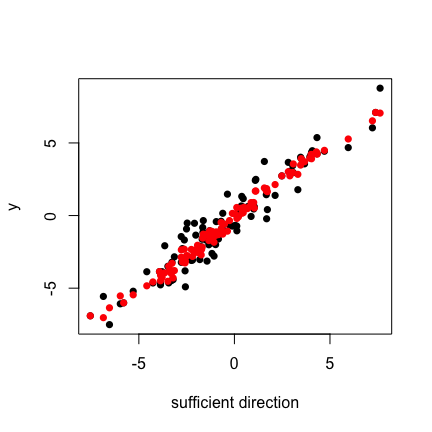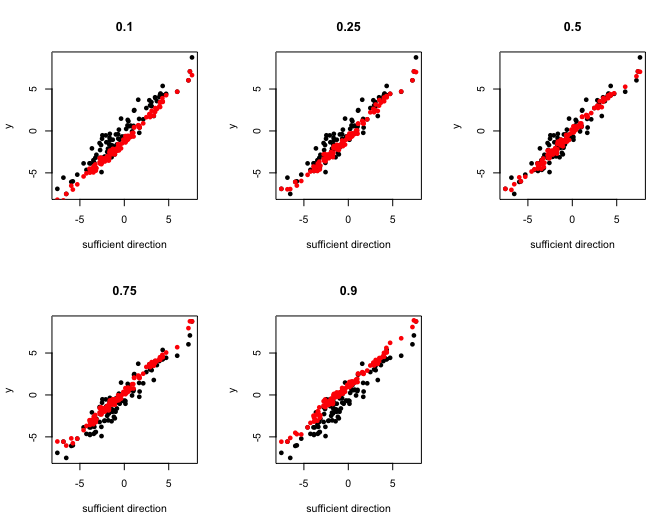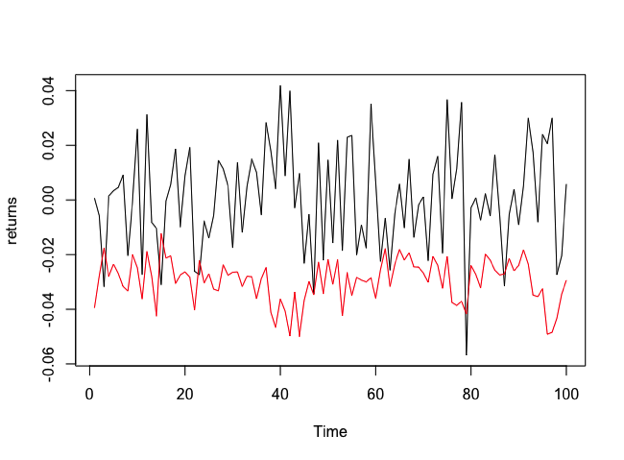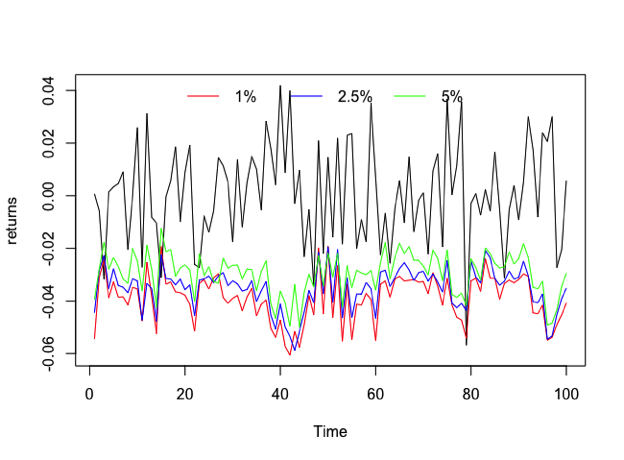# Dimension Reduction Techniques for Conditional Quantiles in R: A Vignette

University of North Carolina at Charlotte

Abstract

While dimension reduction techniques have been well developed, they focus on the entire conditional distribution of the data. However, there are a lot of research areas where extremes are important and require the study of the conditional quantiles. quantdr is an R package that performs dimension reduction to conditional quantiles by determining the directions that span the central quantile subspace (CQS).

# Introduction

Data visualization and non-parametric fitting can become very challenging when data is high-dimensional. This has led to the development of many dimension reduction techniques that focus on the entire conditional distribution of the data. However, when specific aspects of the conditional distribution are of interest, these methods can provide more directions than necessary.

Quantile regression (QR) has received a lot of attention since its inception from Koenker and Bassett (1978) and it has been an attractive tool for research areas where non-central parts of the data are important. Dimension reduction techniques for conditional quantiles have recently made an appearance and are still in development. For some references see Wu et al. (2010), Kong and Xia (2012, 2014), Luo et al. (2014), and Christou and Akritas (2016).

More recently, Christou (2020) proposed a new technique for finding the fewest linear combinations of the predictor variable $$\mathbf{X}$$ that contain all the information about the conditional quantile. To give further intuition, for a univariate response $$Y$$ and a $$p$$-dimensional predictor $$\mathbf{X}$$, the method focuses on finding a $$p \times d_{\tau}$$ matrix $$\mathbf{B}_{\tau}$$, for $$\tau \in (0, 1)$$ and $$d_{\tau} \leq p$$, such that $$Y$$ and $$Q_{\tau}(Y|\mathbf{X})$$ are conditionally independent given $$\mathbf{B}_{\tau}^{\top} \mathbf{X}$$, where $$Q_{\tau}(Y|\mathbf{X})$$ denotes the $$\tau$$th conditional quantile of $$Y$$ given $$\mathbf{X}$$. The space spanned by $$\mathbf{B}_{\tau}$$ is called the $$\tau$$th quantile dimension reduction subspace for the regression of $$Y$$ on $$\mathbf{X}$$. The intersection of all $$\tau$$th dimension reduction subspaces is called the $$\tau$$th central quantile subspace ($$\tau$$-CQS) and is denoted by $$\mathcal{S}_{Q_{\tau}(Y|\mathbf{X})}$$.

The purpose of this vignette is to demonstrate the implementation of the functions appearing in the quantdr package, along with some examples. The main function of the package is cqs which returns the estimated directions of the $$\tau$$-CQS.

# Getting Started

To get started you need to first install the package using the command

install.packages("quantdr")

and then use it during any R session by issuing the command

library(quantdr)

For an overview of the available help files of the package use

help(package = "quantdr")

The package includes the following functions:

• llqr Local linear quantile regression
• llqrcv Cross-Validation for bandwidth selection of local linear quantile regression
• cqs Central quantile subspace
• ValAR Value-at-Risk estimation using the central quantile subspace

To get help on a specific function type help(function.name) or ?function.name for a convenient shorthand. Try

help(cqs)
?cqs

For further examples, refer to the coding examples listed in the documentation for each quantdr function.

# Central quantile subspace

## Overview

The overall goal of the cqs function is to identify the coefficients of the linear combination $$\mathbf{B}_{\tau}^{\top}\mathbf{X}$$ in order to replace the $$p \times 1$$ predictor vector $$\mathbf{X}$$ with the low-dimensional $$d_{\tau} \times 1$$ predictor vector $$\mathbf{B}_{\tau}^{\top}\mathbf{X}$$. To do that, Christou (2020) proved two important results:

• $$\boldsymbol{\beta}_{\tau} \in \mathcal{S}_{Q_{\tau}(Y|\mathbf{X})}$$, where $$\boldsymbol{\beta}_{\tau}$$ is the slope vector from regressing the conditional quantile on $$\mathbf{X}$$, i.e., $\begin{eqnarray*} (\alpha_{\tau}, \boldsymbol{\beta}_{\tau}) = \arg \min_{(a_{\tau}, \mathbf{b}_{\tau})} E \{ Q_{\tau}(Y| \mathbf{A}^{\top} \mathbf{X}) - a_{\tau} - \mathbf{b}_{\tau}^{\top}\mathbf{X}\}^2, \end{eqnarray*}$ and $$\mathbf{A}$$ spans the central subspace (Li 1991). This implies an initial dimension reduction using $$\mathbf{A}$$, making the nonparametric estimation of $$Q_{\tau}(Y|\mathbf{A}^{\top}\mathbf{X})$$ tractable.

• $$E\{Q_{\tau} (Y|U_{\tau})\mathbf{X}\} \in \mathcal{S}_{Q_{\tau}(Y|\mathbf{X})}$$, where $$U_{\tau}$$ is a measurable function of $$\mathbf{B}_{\tau}^{\top}\mathbf{X}$$, provided that $$Q_{\tau}(Y|U_{\tau})\mathbf{X}$$ is integrable.

The above two results imply the following:

1. If the dimension of the $$\tau$$-CQS is known to be one, then we can fit a linear regression model of $$Q_{\tau}(Y|\mathbf{A}^{\top}\mathbf{X})$$ on $$\mathbf{X}$$ and report the slope vector as the basis vector.

2. If the dimension of the $$\tau$$-CQS is known to be greater than one, then we can set $$\boldsymbol{\beta}_{\tau, 0}=\boldsymbol{\beta}_{\tau}$$ as the initial vector and then create more vectors using $$\boldsymbol{\beta}_{\tau,j}=E\{Q_{\tau}(Y | \boldsymbol{\beta}_{\tau, j-1}^{\top}\mathbf{X})\mathbf{X}\}$$, for $$j=1, \dots, p-1$$. In order to obtain linearly independent vectors, we can perform an eigenvalue decomposition on $$\mathbf{V}_{\tau}\mathbf{V}_{\tau}^{\top}$$, where $$\mathbf{V}_{\tau}=(\boldsymbol{\beta}_{\tau, 0}, \dots, \boldsymbol{\beta}_{\tau, p-1})$$ and choose the eigenvectors corresponding to the $$d_{\tau}$$ nonzero eigenvalues.

3. If the dimension of the $$\tau$$-CQS is unknown, we can apply the procedure from 2. Then, we can estimate the dimension $$d_{\tau}$$ using the eigenvalues resulting from the eigenvalue decomposition. Existing techniques such as the Cross-Validation (CV) criterion or the modified-BIC type criterion of Zhu et al. (2010) can be used to determine the structural dimension.

All of the above are incorporated in the cqs function.

## The algorithm at a glance

The first step of the algorithm requires to fit a linear regression model of $$Q_{\tau}(Y|\mathbf{A}^{\top}\mathbf{X})$$ on $$\mathbf{X}$$, which implies the non-parametric estimation of the conditional quantile. The second step of the algorithm relies on estimating an expectation and performing an eigenvalue decomposition. Specifically,

1. We use a dimension reduction technique to estimate the basis matrix $$\mathbf{A}$$ of the central subspace, denoted by $$\widehat{\mathbf{A}}$$, and form the new sufficient predictors $$\widehat{\mathbf{A}}^{\top} \mathbf{X}_{i}$$, $$i=1, \dots, n$$. For this we use the function dr from the package dr.

2. For each $$i=1, \dots, n$$, we use the local linear conditional quantile estimation method of Guerre and Sabbah (2012) to estimate $$Q_{\tau}(Y|\widehat{\mathbf{A}}^{\top} \mathbf{X}_{i})$$. For this we use the llqr of the presented package.

3. We set $$\widehat{\boldsymbol{\beta}}_{\tau}$$ to be $\begin{eqnarray*} (\widehat{\alpha}_{\tau}, \widehat{\boldsymbol{\beta}}_{\tau}) = \arg \min_{(a_{\tau}, \mathbf{b}_{\tau})} \sum_{i=1}^{n} \{\widehat{Q}_{\tau}(Y|\widehat{\mathbf{A}}^{\top}\mathbf{X}_{i}) - a_{\tau} - \mathbf{b}_{\tau}^{\top} \mathbf{X}_{i}\}^2. \end{eqnarray*}$ For this we use the lm function of the stats package.

4. If $$d_{\tau}=1$$ we stop and report $$\widehat{\boldsymbol{\beta}}_{\tau}$$ as the estimated basis vector for $$\mathcal{S}_{Q_{\tau}(Y|\mathbf{X})}$$. Otherwise, we move to Step 5.

5. We set $$\widehat{\boldsymbol{\beta}}_{\tau, 0}=\widehat{\boldsymbol{\beta}}_{\tau}$$, and, for $$j=1, \dots, p-1$$, we
1. form the predictors $$\widehat{\boldsymbol{\beta}}_{\tau, j-1}^{\top} \mathbf{X}_{i}$$, $$i=1, \dots, n$$ and estimate $$Q_{\tau}(Y| \widehat{\boldsymbol{\beta}}_{\tau, j-1}^{\top} \mathbf{X}_{i})$$ using the local linear conditional quantile estimation method. For this we use the llqr function of the presented paper.
2. form $$\widehat{\boldsymbol{\beta}}_{\tau, j} = n^{-1} \sum_{i=1}^{n} \widehat{Q}_{\tau}(Y|\widehat{\boldsymbol{\beta}}_{\tau, j-1}^{\top} \mathbf{X}_{i}) \mathbf{X}_{i}$$.
6. We form the $$p \times p$$ matrix $$\widehat{\mathbf{V}}_{\tau}=(\widehat{\boldsymbol{\beta}}_{\tau, 0}, \dots, \widehat{\boldsymbol{\beta}}_{\tau, p-1})$$ and choose the $$d_{\tau}$$ eigenvectors corresponding to the $$d_{\tau}$$ largest eigenvalues of $$\widehat{\mathbf{V}}_{\tau} \widehat{\mathbf{V}}_{\tau}^{\top}$$. For this we use the eigen function of the base package.

If $$d_{\tau}$$ is unknown, a suggested structural dimension can be estimated using existing techniques such as the CV criterion or the modified-BIC type criterion of Zhu et al. (2010). In terms of the cqs function, the modified-BIC type criterion is utilized and returns a suggested dimension. Note that the user can extract the eigenvalues and apply them to any other existing technique.

## Output of cqs

For the purpose of this section, we simulate data from the homoscedastic single-index model $\begin{eqnarray*} Y=3X_{1}+X_{2}+\epsilon, \end{eqnarray*}$ where $$\mathbf{X}=(X_{1}, \dots, X_{10})^{\top}$$ and the error $$\epsilon$$ are generated according to a standard normal distribution. The $$\tau$$-CQS is spanned by $$(3, 1, 0, \dots, 0)^{\top}$$ for all $$\tau$$. We focus on the estimation of the 0.5-CQS.

set.seed(1234)
n <- 100
p <- 10
tau <- 0.5
x <- matrix(rnorm(n * p), n, p)
error <- rnorm(n)
y <- 3 * x[, 1] + x[, 2] + error

The cqs function proceeds by specifying an $$n \times p$$ design matrix $$\mathbf{X}$$, a vector of the response variable $$Y$$, and a quantile level $$\tau$$. The dimension of the $$\tau$$-CQS, i.e., $$d_{\tau}$$, is optional. Note that for this example $$d_{\tau}=1$$ for every quantile level $$\tau$$.

We now discuss the output of the cqs function.

out1 <- cqs(x, y, tau = tau, dtau = 1)
out1
#> $qvectors #> [,1] #> [1,] 0.952948139 #> [2,] 0.287974471 #> [3,] 0.042667367 #> [4,] 0.038590479 #> [5,] -0.003885815 #> [6,] -0.042769158 #> [7,] 0.028417264 #> [8,] 0.018789922 #> [9,] 0.023914784 #> [10,] 0.045541197 #> #>$dtau
#>  1

When the dimension of the $$\tau$$-CQS is known to be one, the algorithm reports the ordinary least-squares slope vector as the basis vector of the subspace. This is denoted by qvectors. Moreover, the function cqs outputs dtau, which in this case is specified by the user.

When the dimension of the $$\tau$$-CQS is unknown (or known to be greater than one), the algorithm continues, creates more vectors, and performs an eigenvalue decomposition. Therefore, the output includes one more element, the eigenvalues provided by the eigenvalue decomposition and denoted by qvalues.

out2 <- cqs(x, y, tau = tau)
out2
#> $qvectors #> [,1] [,2] [,3] [,4] [,5] [,6] #> [1,] 0.94285965 -0.04373861 0.323724575 0.10473035 0.06290808 -0.04667851 #> [2,] 0.30671396 0.29208998 -0.401758607 -0.16287563 0.15202916 -0.21945183 #> [3,] 0.03101309 -0.28351089 -0.199986929 -0.59193015 -0.45455105 -0.19721619 #> [4,] 0.03394904 -0.16427022 -0.478614025 0.35884778 -0.13384503 0.13125004 #> [5,] -0.00658252 -0.09730212 -0.205810821 -0.08802898 0.75194191 -0.04145494 #> [6,] -0.02433910 0.31968626 0.006386568 -0.65344860 0.37885008 -0.06677676 #> [7,] 0.02707587 0.03398101 0.354491863 -0.21839222 0.12549555 0.77574347 #> [8,] 0.01569753 -0.12261129 -0.358513162 0.23379874 0.04091144 0.40756218 #> [9,] 0.06317845 0.79095189 0.144685863 0.19507455 -0.18329861 -0.20917231 #> [10,] 0.07962450 0.54997345 -0.443034398 -0.38301190 -0.35618445 0.33809519 #> [,7] [,8] [,9] [,10] #> [1,] -0.07376381 -0.16872174 0.008474194 0.14020956 #> [2,] 0.00725682 0.78950190 0.134953357 0.14996842 #> [3,] 0.06903646 -0.25806832 0.566790028 -0.28810369 #> [4,] -0.31360476 -0.09073977 -0.387039672 -0.57510228 #> [5,] -0.39371036 -0.29970274 0.123874984 -0.05495289 #> [6,] 0.47061321 -0.12265678 -0.366212884 -0.45406911 #> [7,] -0.07727430 0.30837138 0.410192154 -0.40082730 #> [8,] 0.74653472 -0.32831788 0.339507633 0.04297342 #> [9,] 0.22776133 -0.25554310 0.624061136 -0.30765592 #> [10,] -0.10565655 -0.04530856 -0.094568566 0.39571912 #> #>$qvalues
#>   9.943967e+01 9.879354e-02 3.285674e-03 4.633843e-04 1.760913e-04
#>   3.195217e-05 1.161632e-05 4.207074e-06 1.322628e-06 1.268057e-10
#>
#> $dtau #>  1 Note that the dimension dtau is correctly estimated by the modified-BIC type criterion. Therefore, this output suggests to take the first eigenvector as the basis vector for the $$\tau$$-CQS. We can extract the direction using out2$qvectors[, 1:out2$dtau] #>  0.94285965 0.30671396 0.03101309 0.03394904 -0.00658252 -0.02433910 #>  0.02707587 0.01569753 0.06317845 0.07962450 If we want to measure the estimation error we can use the angle between the true and the estimated subspaces. This is performed using the subspace function of the pracma package. Note that the angle is measured in radians, and so we divide by $$\pi / 2$$. library(pracma) beta_true <- c(3, 1, rep(0, p - 2)) beta_hat1 <- out1$qvectors
beta_hat2 <- out2$qvectors[, 1:out2$dtau]
subspace(beta_true, beta_hat1) / (pi / 2)
#>  0.06297377
subspace(beta_true, beta_hat2) / (pi / 2)
#>  0.07591763

Note that the estimation error is slightly higher when the dimension of the subspace is unknown and needs to be estimated.

We can continue further and estimate the conditional quantile function. To do this, we first form the new sufficient predictor $$\widehat{\mathbf{B}}_{\tau}^{\top}\mathbf{X}$$ using

newx <- x %*% beta_hat1

We then estimate the conditional quantile using the llqr function of the presented package. The main arguments of the function are: the design matrix, which in this case is $$\widehat{\mathbf{B}}_{\tau}^{\top}\mathbf{X}$$, the vector of the response variable $$Y$$, and the quantile level $$\tau$$. The rest of the arguments, i.e., the bandwidth $$h$$, the method for the estimation of $$h$$, and the single observation $$x_0$$ for which to perform the estimation, are optional. If $$h$$ is not specified, then it will be determined using either the rule-of-thumb bandwidth of Yu and Jones (1998) or the CV criterion; the default choice is the rule-of-thumb. However, the user needs to be careful about the bandwidth selection. When the dimension of the predictor variable is large compared to the sample size, local linear fitting meets the ‘curse of dimensionality’ problem. In situations like that, the bandwidth selected by the rule-of-thumb or the CV criterion might be small and cause the function to fail. For these cases, we advice the user to pre-specify the bandwidth in the function. Finally, if $$x_0$$ is not specified, the estimation will be performed on the entire design matrix. For this example we use

qhat1 <- llqr(newx, y, tau)
qhat1
#> $ll_est #>  -4.29612236 0.50206070 2.83802778 -6.90420017 0.19579698 1.85561955 #>  -2.31913140 -1.33434436 -1.72001737 -3.20272158 -1.28031021 -3.94174571 #>  -3.49390807 0.52131417 2.73413065 0.56630321 -2.36093711 -3.12115648 #>  -2.51407640 6.52189600 -0.08583814 -2.33251792 0.55809983 2.13418429 #>  -0.34625794 -4.56943348 1.68280128 -4.83741324 -0.91380392 -3.26092075 #>  4.29466772 -1.08891160 -3.99935145 -1.31242281 -6.35220726 -4.49313490 #>  -7.03023081 -5.53238392 0.11381859 -2.35896143 4.21208577 -2.60786133 #>  -2.04607770 -1.12522131 -2.76437352 -2.77165266 -1.55178162 -4.52444343 #>  -1.83495363 -1.63030447 -6.01074528 -1.51250554 -2.17197891 -3.85853540 #>  -1.07434001 2.96517288 4.49027277 -3.72385461 3.95256798 -4.11844704 #>  1.69068143 7.09879779 -0.58651720 -2.81532043 0.15631061 5.27102863 #>  -2.71082703 3.91064983 3.70356704 2.73121453 1.64619818 -1.30946960 #>  -1.27896874 0.55501360 7.05577045 -1.05247670 -5.45933552 0.89422383 #>  0.89854628 -1.36919251 -3.28789275 -1.27642763 -3.78906596 0.14684430 #>  3.47472770 4.20733501 3.01834505 -2.32795975 -0.19731735 -4.25561959 #>  -0.47404473 -2.57259595 4.22777744 3.91939381 0.47869246 1.89990559 #>  -2.87174638 1.69526276 3.55421064 4.38360715 #> #>$h
#>  0.3526179

The output consists of the estimation of the conditional quantile function at each point of the design matrix, i.e., $$\widehat{Q}_{\tau}(Y|\widehat{\mathbf{B}}_{\tau}^{\top} \mathbf{X}_{i})$$, $$i=1, \dots, n$$, and the estimation of the bandwidth using the rule-of-thumb. For illustration purposes, we repeat the above using the CV criterion for the bandwidth selection.

qhat2 <- llqr(newx, y, tau, method = "CV")
qhat2
#> $ll_est #>  -4.16471702 0.42243692 2.92565950 -6.90420017 0.13404652 1.85561955 #>  -2.37072469 -1.34044965 -1.74117469 -3.22002253 -1.28563104 -3.81894259 #>  -3.53049520 0.44056678 2.83018199 0.48293029 -2.41430029 -3.15966390 #>  -2.57392284 6.65279536 -0.13115259 -2.38467794 0.47520565 2.13529423 #>  -0.37101672 -4.30454499 1.62995552 -4.65447360 -0.91380392 -3.27669481 #>  4.35903141 -1.08469511 -3.86885124 -1.31820985 -6.35093348 -4.23891907 #>  -7.04312759 -5.49387034 0.05685232 -2.41224096 4.23569199 -2.67233348 #>  -2.09085856 -1.12013049 -2.83530198 -2.84288140 -1.56852396 -4.26584816 #>  -1.87138464 -1.64910747 -6.01074528 -1.52119732 -2.22173910 -3.74999646 #>  -1.07047439 3.04250102 5.05306616 -3.64641578 3.88369939 -3.99491726 #>  1.63802186 7.09879779 -0.59906765 -2.88835051 0.09686454 5.54979008 #>  -2.77954663 3.83234757 3.56330169 2.82750218 1.59248756 -1.31521376 #>  -1.28427010 0.47229953 7.07269696 -1.04913754 -5.41494071 0.87880733 #>  0.88309542 -1.37580371 -3.30295918 -1.28169210 -3.69008814 0.08795068 #>  3.36253416 4.21394754 3.09136422 -2.37992680 -0.22964427 -4.12600955 #>  -0.49231026 -2.63561335 4.25858264 3.80649004 0.40043244 1.89990559 #>  -2.94710410 1.64271143 3.43847962 4.50888583 #> #>$h
#>  0.5981072

If $$h$$ is pre-specified, then the output reports the value given by the user, i.e.,

qhat3 <- llqr(newx, y, tau, h = 1)
qhat3
#> $ll_est #>  -4.10733119 0.41575048 2.82984989 -6.96560477 0.12867419 1.80232825 #>  -2.31913140 -1.33238865 -1.71846335 -3.13228281 -1.27829822 -3.78217584 #>  -3.43094360 0.43379773 2.73390739 0.47596820 -2.36093711 -3.07602690 #>  -2.51407640 6.86316722 -0.13531649 -2.33251792 0.46827876 2.07701731 #>  -0.37942098 -4.33565856 1.58076620 -4.56941194 -0.91141020 -3.18679900 #>  4.36291723 -1.08003138 -3.83145286 -1.31044427 -6.38394707 -4.27831201 #>  -7.11408028 -5.47538775 0.05183175 -2.35896143 4.23499991 -2.60848998 #>  -2.04607770 -1.11499607 -2.76483932 -2.77211089 -1.55005238 -4.30757720 #>  -1.83495363 -1.62865701 -6.01074528 -1.51073539 -2.17197891 -3.74999646 #>  -1.06599957 2.94726045 5.17473676 -3.64641578 3.87772477 -3.95592341 #>  1.58868591 7.09879779 -0.60462800 -2.81573322 0.09166164 5.80181928 #>  -2.71134854 3.82487019 3.55953216 2.73121453 1.54397932 -1.30748798 #>  -1.27695535 0.46538589 7.07218396 -1.04494614 -5.38301842 0.86579411 #>  0.87005822 -1.36727309 -3.21206415 -1.27441160 -3.69008814 0.08278840 #>  3.35565465 4.21259803 2.99636162 -2.32795975 -0.23981152 -4.08535663 #>  -0.49920193 -2.57326128 4.25858264 3.79825597 0.39384627 1.84580928 #>  -2.87210046 1.59329025 3.43277654 4.52152403 #> #>$h
#>  1

To illustrate the median regression, compared with the original data, we can use

true_dir <- x %*% beta_true

plot(true_dir, y, xlab = "sufficient direction", ylab = "y", pch = 16)
points(true_dir, qhat1$ll_est, pch = 16, col = 'red')The same procedure can be performed for multiple quantile levels. For example, we estimate the $$\tau$$-CQS for $$\tau= 0.1, 0.25, 0.5, 0.75, 0.9$$. taus <- c(0.1, 0.25, 0.5, 0.75, 0.9) out3 <- matrix(0, p, length(taus)) for (i in 1:length(taus)) { out3[, i] <- cqs(x, y, tau = taus[i], dtau = 1)$qvectors
}
out3
#>               [,1]         [,2]         [,3]        [,4]         [,5]
#>  [1,]  0.955128387  0.953563098  0.952948139  0.95432205  0.957157424
#>  [2,]  0.282221145  0.286814969  0.287974471  0.28469153  0.275583662
#>  [3,]  0.040025231  0.040124118  0.042667367  0.04252055  0.039230711
#>  [4,]  0.037794516  0.037393312  0.038590479  0.03742298  0.037849461
#>  [5,] -0.002145479 -0.002710592 -0.003885815 -0.00284509  0.002339282
#>  [6,] -0.049903668 -0.047392988 -0.042769158 -0.05199378 -0.053432303
#>  [7,]  0.029549555  0.029730897  0.028417264  0.02857128  0.032300729
#>  [8,]  0.016985520  0.017341603  0.018789922  0.01678999  0.017397007
#>  [9,]  0.015690746  0.019725351  0.023914784  0.01175831  0.009852825
#> [10,]  0.033877447  0.040239263  0.045541197  0.03261534  0.025062461

Similarly, we can plot the data along with the estimated conditional quantile functions for the various quantile levels.

newx <- x %*% out3

par(mfrow=c(2,3))
qhat_tau <- as.null()
for (i in 1:length(taus)) {
plot(true_dir, y, xlab = "sufficient direction", ylab = "y", main = taus[i], pch = 16)
qhat_tau <- llqr(newx[, i], y, tau = taus[i])\$ll_est
points(true_dir, qhat_tau, pch = 16, col = "red")
}
par(oldpar)# Value-at-Risk Estimation

## Overview

Value-at-risk (VaR) is an important financial quantity directly related to the QR framework. Specifically, for $$\{r_{t}\}_{t=1}^{n}$$ a time series of returns, the $$\tau$$th VaR at time $$t$$, denoted by $$VaR_{\tau}(t)$$, is the smallest number for which $$P\{r_{t} < -VaR_{\tau}(t) | \mathcal{F}_{t-1}\} = \tau$$, where $$\mathcal{F}_{t-1}$$ denotes the information set at time $$t-1$$. Therefore, $$-VaR_{\tau}(t)$$ is the $$\tau$$th conditional quantile of $$r_{t}$$, and we can write $$Q_{\tau}(r_{t} | \mathcal{F}_{t-1}) = -VaR_{\tau}(t)$$.

Christou and Grabchak (2019) used the methodology proposed by Christou (2020) to estimate VaR and compare it with existing commonly-used methods. The estimation of $$VaR_{\tau}(t)$$ at time $$t$$ reduces to the estimation of $$Q_{\tau}(r_{t} | \mathcal{F}_{t-1})$$, which can be performed using the cqs and llqr functions of the presented package. Specifically, let $$r_{t}$$ denote a daily return (usually log-return) and $$\mathbf{r}_{t}$$ denote a $$p$$-dimensional vector of covariates consisting of the $$p$$ past returns, i.e., $$\mathbf{r}_{t,p} = (r_{t-1},\dots, r_{t-p})^{\top}$$. We want to estimate $$Q_{\tau}(r_{t} | \mathbf{r}_{t,p})$$ at time $$t$$ by assuming that there exists a $$p \times d_{\tau}$$ matrix $$\mathbf{B}_{\tau}$$, $$d_{\tau} \leq p$$, such that $$Q_{\tau}(r_{t} | \mathbf{r}_{t,p}) = Q_{\tau}(r_{t} | \mathbf{B}_{\tau}^{\top}\mathbf{r}_{t,p})$$. This implies that $$\mathbf{B}_{\tau}^{\top}\mathbf{r}_{t,p}$$ contains all the information about $$r_{t}$$ that is available from $$Q_{\tau}(r_{t} | \mathbf{r}_{t,p})$$. Once $$\mathbf{B}_{\tau}$$ is estimated using the cqs function, the sufficient predictors $$\widehat{\mathbf{B}}_{\tau}^{\top}\mathbf{r}_{t,p}$$ are formed and $$Q_{\tau}(r_{t} | \widehat{\mathbf{B}}_{\tau}^{\top}\mathbf{r}_{t,p})$$ is estimated using the llqr function.

## Output of ValAR

For an illustration of VaR estimation, we use the edhec data set available in the PerformanceAnalytics package in R. The data set includes the EDHEC composite hedge fund style index returns.

library(PerformanceAnalytics)
data(edhec, package = "PerformanceAnalytics")
#>            Convertible Arbitrage CTA Global Distressed Securities
#> 1997-01-31                0.0119     0.0393                0.0178
#> 1997-02-28                0.0123     0.0298                0.0122
#> 1997-03-31                0.0078    -0.0021               -0.0012
#> 1997-04-30                0.0086    -0.0170                0.0030
#> 1997-05-31                0.0156    -0.0015                0.0233
#> 1997-06-30                0.0212     0.0085                0.0217
#>            Emerging Markets Equity Market Neutral Event Driven
#> 1997-01-31           0.0791                0.0189       0.0213
#> 1997-02-28           0.0525                0.0101       0.0084
#> 1997-03-31          -0.0120                0.0016      -0.0023
#> 1997-04-30           0.0119                0.0119      -0.0005
#> 1997-05-31           0.0315                0.0189       0.0346
#> 1997-06-30           0.0581                0.0165       0.0258
#>            Fixed Income Arbitrage Global Macro Long/Short Equity
#> 1997-01-31                 0.0191       0.0573            0.0281
#> 1997-02-28                 0.0122       0.0175           -0.0006
#> 1997-03-31                 0.0109      -0.0119           -0.0084
#> 1997-04-30                 0.0130       0.0172            0.0084
#> 1997-05-31                 0.0118       0.0108            0.0394
#> 1997-06-30                 0.0108       0.0218            0.0223
#>            Merger Arbitrage Relative Value Short Selling Funds of Funds
#> 1997-01-31           0.0150         0.0180       -0.0166         0.0317
#> 1997-02-28           0.0034         0.0118        0.0426         0.0106
#> 1997-03-31           0.0060         0.0010        0.0778        -0.0077
#> 1997-04-30          -0.0001         0.0122       -0.0129         0.0009
#> 1997-05-31           0.0197         0.0173       -0.0737         0.0275
#> 1997-06-30           0.0231         0.0198       -0.0065         0.0225

To estimate the one-step ahead VaR we need a vector of returns in a standard chronological order. For this, we will select the ‘CTA Global Distressed’ as a vector of returns. The ValAR function proceeds by specifying the vector of returns $$y$$, the number $$p$$ of past observations to be used as the predictor variables, the quantile level $$\tau$$, a moving window for the number of observations to be used for fitting the model, and a logical operator to indicate whether the returns are given in a standard chronological order (oldest to newest) or not. If a moving window is not specified, then the default value of min(250, n - p) will be used to estimate the conditional quantile. Note that, typical values for a moving window correspond to one or two years of returns. A moving window will be useful if the number of observations is large and old returns will not be of much value to the prediction. Moreover, if the number of observations is large, a moving window will also speed up the algorithm, since the number of observations to be used will be smaller. Finally, if the returns are in reversed chronological order, then the user should either reverse the vector of returns or indicate that using the chronological option of the function.

For this example, we will use $$p = 5$$, $$\tau = 0.05$$, and will not specify any moving window so that the default value of min(250, 275 - 5) = 250 will be used. Also, the returns are given in standard chronological order and, since this is the default setting, we do not specify anything for chronological. Note that, the last day of returns is 2019-11-30 and therefore, we are predicting the 5% VaR for 2019-12-31.

y <- as.vector(edhec[, 2])
n <- length(y)
p <- 5
tau <- 0.05
ValAR(y, p = p, tau = tau)
#>  -0.03154725

If we want to compare this number with the 5% VaR estimated by the historical method, we can use

VaR(y, 0.95, method = 'historical')
#>         [,1]
#> VaR -0.03163

For illustration purposes, we will use the last 100 observations as a testing data set for one-step ahead VaR estimation and the rest of the observations as historical data. Specifically, for each of the time points, $$t$$, of the observations not included in the historical data, we estimate the model based on all of the data up to time $$t-1$$ and report the 5% VaR.

size <- 100

VaRest <- as.null(size)
for (i in 1:size){
VaRest[i] <- ValAR(y[1:(n - size + i - 1)], p, tau)
}

To visualize the results, we can plot the time series of returns with the one-step ahead 5% VaR forecasts.

plot.ts(y[(n - size + 1):n], ylim = range(y[(n - size + 1):n], VaRest), ylab = 'returns')
lines(VaRest, col = 'red')Moreover, we can calculate the proportion of exceptions, i.e., the number of times $$r_{t} < -\widehat{VaR}_{\tau}(t)$$ for each time point $$t$$ of the last 100 observations. This number should be a reasonable estimate for $$\tau$$. In this case we can see that the estimated proportion is actually 0.05.

sum(y[(n - size + 1):n] < VaRest) / size
#>  0.05

We can repeat the above procedure for multiple quantile levels, i.e., for $$\tau= 0.01, 0.025, 0.05$$.

taus <- c(0.01, 0.025, 0.05)
VaRest <- matrix(0, size, length(taus))
for (i in 1:size) {
for (j in 1:length(taus)) {
VaRest[i, j] <- ValAR(y[1:(n - size + i - 1)], p, taus[j])
}
}

# plots
plot.ts(y[(n - size + 1):n], ylim = range(y[(n - size + 1):n], VaRest), ylab = 'returns')
lines(VaRest[, 1], col = 'red')
lines(VaRest[, 2], col = 'blue')
lines(VaRest[, 3], col = 'green')
legend('top', legend = c("1%", "2.5%", "5%"), col = c("red", "blue", "green"),
lty=1, cex=1, horiz = T, bty = "n")# proportion of exceptions
sum(y[(n - size + 1):n] < VaRest[, 1]) / size
#>  0.03
sum(y[(n - size + 1):n] < VaRest[, 2]) / size
#>  0.03
sum(y[(n - size + 1):n] < VaRest[, 3]) / size
#>  0.05

We can see that the estimated proportion of exceptions are very close to the true values of 0.01, 0.025, and 0.05.

# Conclusion

This vignette provides a brief introduction to the R package quantdr and presents a tutorial on how to implement the basic function cqs. Performing dimension reduction techniques to conditional quantiles is an active research topic and therefore updates and new functions will be incorporated into forthcoming versions of the package. For this reason, this document will be updated accordingly and will be made available through the package. Suggestions and recommendations from the users will be highly appreciated and welcomed. This package is also available through GitHub. Feel free to contact the author at .